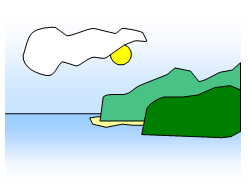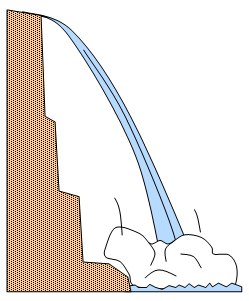# Heat energy

The jam in a sponge pudding stays hotter for longer than the pudding around it
Water is a very good coolant for use in engines
Saucepans are made of materials with a low mass if possible
It takes a long time to heat up enough water to have a hot bath
Houses built with thick stone walls keep warm in winter and stay cool in summer
People used to warm up heavy china teapots before putting the hot water and tea in them
Storage radiators are used to retain heat energy for use later in the day
You can put out a candle flame (temperature 800 oC) with moist fingers without pain but putting your hand into a bowl of boiling water (100 oC) would hurt a lot!
Electronic circuits are built with "heat sinks"
When you run "hot" water into a basin it cools as soon as it touches the material of the basin
Liquid sodium is used as a coolant in some nuclear reactorsAll these facts are related to heat energy and to a quantity known as specific heat capacity and we can explain them by looking more closely at heat energy.
Over the past few centuries scientists have put forward some very strange theories about the nature of heat. One of these was that heat was some sort of a fluid that you added to a body to make it hot and took away from a body to cool it down.

However, during the last century two men, Rumford and Joule, both proposed the idea that heat was related to energy. When heat energy passes into a body it increases the internal energy of the body. Rumford demonstrated this in some well-known experiments in cannon boring and Joule showed that the friction generated between a paddle wheel and some water would heat up the water.

We can summarise these results as:

To heat up a substance requires energy. This energy increases the internal energy of the substance by increasing the kinetic energy of its molecules and so the temperature of the substance rises

## Heat capacity and specific heat capacity

The amount of heat energy needed to change the temperature of a substance depends on:
(a) what the substance is;
(b) how much of it is being heated;
(c) what rise in temperature occurs.

The heat energy needed to raise the temperature of an object by 1 K is called the HEAT CAPACITY of the object.

However, a rather more useful quantity is the heat energy needed for 1 kg only.

The SPECIFIC HEAT CAPACITY of a substance is the heat needed to raise the temperature of 1 kg of the substance by 1K (or by 1oC).

Specific heat capacity is given the symbol c. The units for c are J/(kg K) or J/(kgoC).
The values for the specific heat capacities of some common substances are given in the following table:

 Substance Specific heat capacity (J/(kgK) Substance Specific heat capacity (J/(kgK) Water 4200 Aluminium 913 Cast iron 500 Brick 840 Copper 385 Concrete 880 Lead 126 Marble 880Remember that substances with high specific heat capacities take a lot of heat energy and therefore a long time to heat up and also a long time to cool down.

One interesting effect is the way in which the land heats up quicker than the sea - the specific heat capacity of sea water is greater than that of the land and so more heat energy is needed to heat it up by the same amount as the land and so it takes longer. It also takes longer to cool down.

The heat energy needed to raise the temperature of m kg of a substance of specific heat capacity c by a certain temperature difference is given by the equation:

Heat energy = mass x specific heat capacity x temperature change

If the object cools then it gives out heat energy and if it heats up it takes in heat energy.

Example problems
1. How much heat energy is needed to raise the temperature of 3 kg of copper by 6 K?
(Specific heat capacity of copper = 385 J/(kg K)
Heat energy = mass x specific heat capacity x temperature change = 3 x 385x 6 = 6930J

2. What is the rise in temperature of 5 kg of water if it is given 84 000 J of heat energy?
Specific heat capacity of water = 4200 J/(kg K).
Heat energy input = 84000 = 5x4200x temperature rise
Temperature rise = 84000/[5x4200] = 4 K

3. How much heat is lost by 3 kg of lead when it cools from 1000 oC to 200 oC?
Specifc heat capacity of lead = 126 J/(kg K)
Heat energy given out = 3x126x80 = 30240J

4. A heater of 800W is use to heat a 600 g cast iron cooker plate.
How long will it take to raise the temperature of the plate by 200 oC?
Specific heat capacity of iron = 500 J/(kg K)
Heat energy needed = 0.6x500x200 = 60 000J
Time needed = 60 000/800 = 75 s = 1 minute 15 s

## Conversion of mechanical energy to heatWhen an object falls to the ground, the potential energy that it had at the top is converted to kinetic energy that finally becomes heat energy.
Assuming no loss of energy to any other forms we can work out the rise in temperature of water falling over a high waterfall.

Example problem
Height of waterfall 84 m.
Consider a mass of water m kg
Specific heat capacity of water = 4200 J/(kgoC).
Gravitational field of the Earth = 10 N/kg
Potential energy lost = Heat energy gained
m x10 x 84 = m x 4200 x temperature rise
Temperature rise = [10 x 84]/4200 = 0.2oC.

There must be no residual kinetic energy of spray and no sound must be made! (Clearly not true but it is the best we can do without making the problem very difficult).

This conversion of gravitational potential energy into heat energy can be used in the laboratory to measure the specific heat capacity of lead shot.

Example problem
Length of cardboard tube = 1 m
Mass of lead shot = m kg
The tube is upended ten times giving a total height fallen of 10m
Temperature rise 0.80 oC
Heat energy gained by the lead shot = potential energy lost by the lead shot
Expressed as a formula:   m x specific heat capacity x 0.8 =m x10x10
Therefore specific heat capacity of lead = 10x10/0.8 = 125 J/(kgoC)

A VERSION IN WORD IS AVAILABLE ON THE SCHOOLPHYSICS USB

© Keith Gibbs 2020